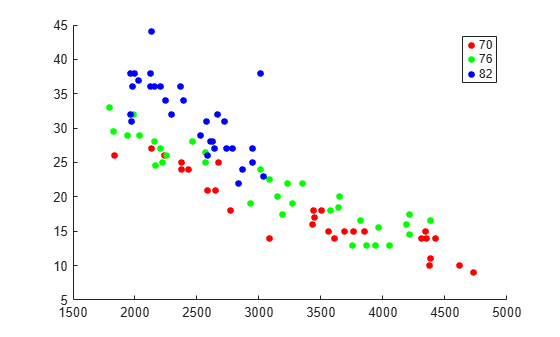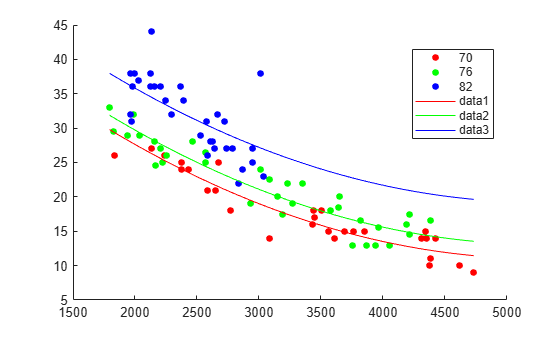# feval

Predict responses of linear regression model using one input for each predictor

## Syntax

``ypred = feval(mdl,Xnew1,Xnew2,...,Xnewn)``

## Description

example

````ypred = feval(mdl,Xnew1,Xnew2,...,Xnewn)` returns the predicted response of `mdl` to the new input predictors `Xnew1,Xnew2,...,Xnewn`.```

## Examples

collapse all

Fit a mileage model to the `carsmall` data set, including the `Year` categorical predictor. Superimpose fitted curves on a scatter plot of the data.

Load the data set and fit the model.

```load carsmall tbl = table(MPG,Weight); tbl.Year = categorical(Model_Year); mdl = fitlm(tbl,'MPG ~ Year + Weight^2');```

Create a scatter plot of `MPG` versus `Weight`, grouped by `Year`.

`gscatter(tbl.Weight,tbl.MPG,tbl.Year);`Plot curves of the model predictions for the various years and weights by using `feval`.

```w = linspace(min(tbl.Weight),max(tbl.Weight))'; line(w,feval(mdl,w,'70'),'Color','r') line(w,feval(mdl,w,'76'),'Color','g') line(w,feval(mdl,w,'82'),'Color','b')```## Input Arguments

collapse all

Linear regression model object, specified as a `LinearModel` object created by using `fitlm` or `stepwiselm`, or a `CompactLinearModel` object created by using `compact`.

New predictor values, specified as a vector, matrix, table, or dataset array.

• If you pass multiple inputs `Xnew1,Xnew2,...,Xnewn` and each includes observations for one predictor variable, then each input must be a vector. Each vector must have the same size. If you specify a predictor variable as a scalar, then `feval` expands the scalar argument into a constant vector of the same size as the other arguments.

• If you pass a single input `Xnew1`, then `Xnew1` must be a table, dataset array, or matrix.

• If `Xnew1` is a table or dataset array, it must contain predictors that have the same predictor names as in the `PredictorNames` property of `mdl`.

• If `Xnew1` is a matrix, it must have the same number of variables (columns) in the same order as the predictor input used to create `mdl`. Note that `Xnew1` must also contain any predictor variables that are not used as predictors in the fitted model. Also, all variables used in creating `mdl` must be numeric. To treat numerical predictors as categorical, identify the predictors using the `'CategoricalVars'` name-value pair argument when you create `mdl`.

Data Types: `single` | `double` | `table`

## Output Arguments

collapse all

Predicted response values at `Xnew1,Xnew2,...,Xnewn`, returned as a numeric vector.

## Tips

• A regression object is, mathematically, a function that estimates the relationship between the response and predictors. The `feval` function enables an object to behave like a function in MATLAB®. You can pass `feval` to another function that accepts a function input, such as `fminsearch` and `integral`.

• `feval` can be simpler to use with a model created from a table or dataset array. When you have new predictor data, you can pass it to `feval` without creating a table or matrix.

## Alternative Functionality

• `predict` gives the same predictions as `feval` by using a single input argument containing all predictor variables, rather than multiple input arguments with one input for each predictor variable. `predict` also gives confidence intervals on its predictions.

• `random` predicts responses with added noise.